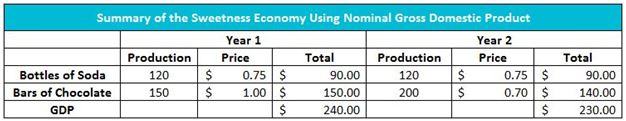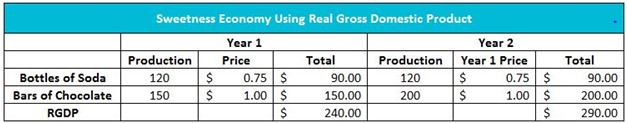# Real Gross Domestic Product (RGDP)

## Definition of Real Gross Domestic Product:

Real gross domestic product is the gross domestic product (GDP) after adjusting for inflation.

## Detailed Explanation:

A change in either the amount produced or price level will affect GDP. Economists adjust for inflation to measure the true change in production. GDP can be measured as both “real” and “nominal,” although nominal GDP is most common. To illustrate the distinction and potential confusion, consider Sweetness’s economy. Sweetness is a tiny country that produces only soft drinks and chocolate. In Year 1, the economy produced 120 bottles of soft drinks and 150 bars of chocolate. The price of a bottle of soft drink was \$0.75, and the price of a bar of chocolate was \$1.00. Sweetness’s GDP equaled \$240 in Year 1. This is calculated by adding the market value of the soft drinks produced (120 x \$0.75 = \$90) and the market value of chocolate produced (150 x \$1.00 = \$150).

Assume the cost of producing chocolate bars is less in Year 2. The forces of supply and demand decreased the price of a bar to \$0.70. The quantity demanded increased to 200 bars at \$0.70. To meet the added demand, production was increased by 33 percent to 200 bars. Therefore, Sweetness’s GDP decreased to \$230 in Year 2 even though production of chocolate bars increased, and the production of soda remained unchanged. Table 1 summarizes the calculation of nominal GDP in Sweetness in Years 1 and 2.

Table 1How can this happen? GDP is a measure of production, and clearly the economy produces more goods in Year 2. However, an economist would calculate a decreased GDP after multiplying the price and quantity of the goods produced because the price of chocolate bars is lower. Even though the actual production of goods increases in Year 2, the lower price of chocolate has a greater impact on GDP than the increase in production. To measure the real increase in production, economists hold the price of the goods and services constant. Table 2 shows that the real gross domestic product increases to \$290 when using Year 1 prices. The real gross domestic product (RGDP) is the gross domestic product adjusted for changes in prices. Nominal gross domestic product uses the prices of the same year GDP is measured. Sweetness has a nominal gross domestic product of \$230 in Year 2. Unless otherwise stated, GDP figures are stated in nominal terms.

Table 2In economics, it is useful to measure the relationship between the GDP and the RGDP to determine what portion of the increase in nominal GDP is caused by a change in production and what portion is caused by a change in prices.

## Dig Deeper With These Free Lessons:

Gross Domestic Product - Measuring an Economy's Performance
Causes of Inflation
Inflation